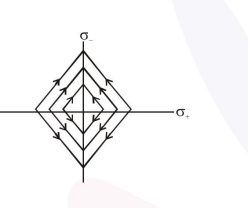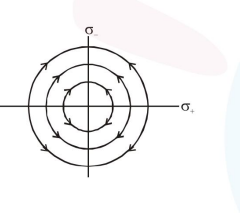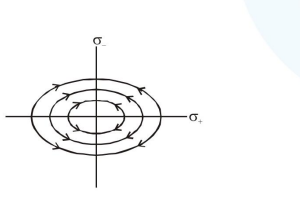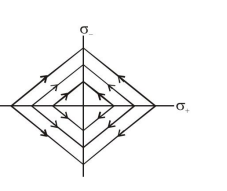# Two charged thin infinite plane sheets of uniform surface charge density

Question:

Two charged thin infinite plane sheets of uniform surface charge density $\sigma_{+}$and $\sigma_{-}$ where $\left|\sigma_{+}\right|>\left|\sigma_{-}\right|$intersect at right angle. Which of the following best represents the electric field lines for this system :

1.2.3.4.Correct Option: 1

Solution:

Thin infinite uniformly charged planes produces uniform electric field therefore option 2 and option 3 are obviously wrong.

And as positive charge density is bigger in magnitude so its field along $\mathrm{Y}$ direction will be bigger than field of negative charge in $X$ direction and this is evident in option 1 so it is correct.